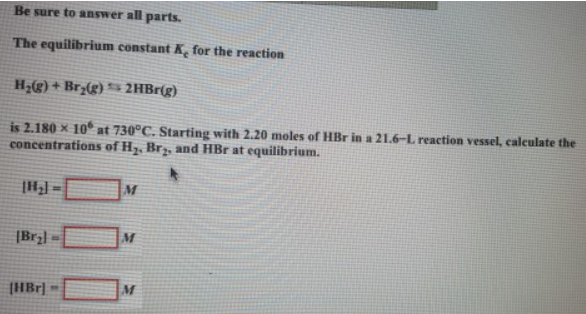# Problem: Be sure to answer all parts.The equilibrium constant Kc for the reaction H2 (g) + Br2 (g) ⇋ 2HBr (g) is 2.180 x 106 at 730°C. Starting with 2.20 moles of HBr in a 21.6-L reaction vessel, calculate the concentrations of H2, Br2 and HBr at equilibrium.

###### FREE Expert Solution
93% (124 ratings)###### Problem Details

Be sure to answer all parts.

The equilibrium constant Kc for the reaction

H2 (g) + Br2 (g) ⇋ 2HBr (g)

is 2.180 x 106 at 730°C. Starting with 2.20 moles of HBr in a 21.6-L reaction vessel, calculate the concentrations of H2, Br2 and HBr at equilibrium.